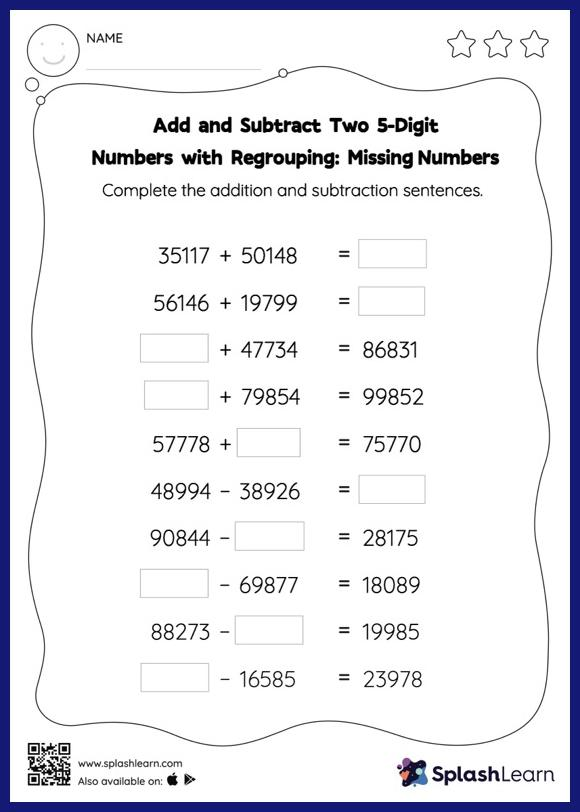# Add and Subtract Two 5-Digit Numbers with Regrouping: Missing Numbers Worksheet

Home > Add and Subtract Two 5-Digit Numbers with Regrouping: Missing NumbersGive your little one the opportunity to dive deeper into addition and subtraction with this add and subtract two 5-digit numbers with regrouping worksheet. In this worksheet, students must find the missing number using the relationship between addition and subtraction. To solve add and subtract two 5-digit numbers with regrouping worksheet, they must also regroup the numbers. This worksheet is about practicing with the horizontal format in which numbers are written side by side. To develop flexibility with numbers and operations, students need to have enough practice in this format and not just rely on the vertical/column method.